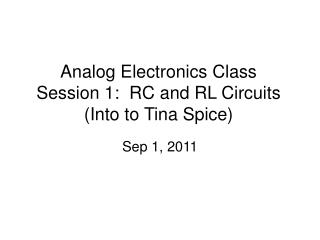DownloadDownload PresentationAnalog Electronics Class Session 1: RC and RL Circuits (Into to Tina Spice)

# Analog Electronics Class Session 1: RC and RL Circuits (Into to Tina Spice)

Download Presentation## Analog Electronics Class Session 1: RC and RL Circuits (Into to Tina Spice)

- - - - - - - - - - - - - - - - - - - - - - - - - - - E N D - - - - - - - - - - - - - - - - - - - - - - - - - - -
##### Presentation Transcript

1. Analog Electronics ClassSession 1: RC and RL Circuits(Into to Tina Spice) Sep 1, 2011

2. Intro to the Class Class 1: Review RC, and RL Circuits Class 2: Ideal op-amp, Analysis Techniques Class 3: Vosi, Vcm, CMRR, PSRR, Class 4: Aol, Ib, Drift, Output swing Class 5: Power…. Class 6: Noise… This will probably change

3. Goal of Class • Intuitive view of analog electronics • Quick analysis by inspection • Use of spice tools • Cover practical considerations • Example: Capacitor temperature drift, voltage coefficients • Fill in gaps in knowledge

4. Capacitor Transient Analysis

5. Capacitor Basics

6. Constant Voltage -> Zero Current

7. Constant Current -> Voltage Changes Linearly

8. Instantaneous changes on capacitor voltage are not possible! This would create infinite current.

9. Application – Slew Rate

10. Equations for RC Charging / Discharging

11. DC RC Circuits For Capacitors

12. Application – Charging Input cap A simple RC is sometimes used to condition the input to an A/D converter. The table shows the delay required to settle to ½ bit.

13. Tina Spice – Transient Analysis Transient Analysis: Looks at signals vs. time. Set the Source: In this example we will use a step input to change the input from 0V to 1V

14. Press the button to edit the signal. Choose the amplitude and start time. In this example 1V and 1mS.

15. Run the transient analysis.

16. Set the time range for the analysis.

17. This is the result Use separate curves

18. Click on the axis and adjust as desired.

19. Use the curser to check the specific points on the curve. In this case 1 time constant yields 0.625V as expected.

20. Capacitor AC Analysis

21. Capacitor AC

22. Capacitance at dc and High Frequency Open at Low Frequency and DC Short at High Frequency

23. Low Pass Filter

24. High Pass Filter

25. Voltage Divider Equation for Resistors

26. Voltage Divider for RC circuit • At low frequencies the output is equal to the input. • At High frequencies the output is zero (shorted)

27. Decibels (dB) • NdB = 20 log (Gain) = 20 log (Vout / Vin) • 20Log(1) = 0, • 20Log(10) = 20 • 20Log(100) = 40 • 20Log(0.1) = -20 • 20Log(0.01) = -40 • Log(0) is not defined • Gain = 10(Ndb/20)

28. After pole decrease Gain by 20db/dec After zero increase Gain by 20db/dec One decade before and after pole phase decreases by -45o/decade One decade before and after zero phase increases by 45o/decade Bode Plot

29. Simulating AC Transfer Characteristics

30. Inductor Transient Analysis

31. Inductor Basics

32. DC RL Circuits For Inductors

33. Equations for RL Charging / Discharging

34. Transient Simulation for RL Circuits Change the prosperities of the switch to close and open to demonstrate charge / discharge.

35. S1 Closed -- Initial Voltage During Charge through R1 S1 Open – Discharge through R2

36. Application – Relay Coil

37. Inductor AC Analysis

38. Inductor AC

39. Inductance at dc and High Frequency Short at Low Frequency and DC Open at High Frequency

40. Voltage Divider for LR circuit • At low frequencies the output is zero. • At High frequencies the output is equal to the input

41. High Pass Filter